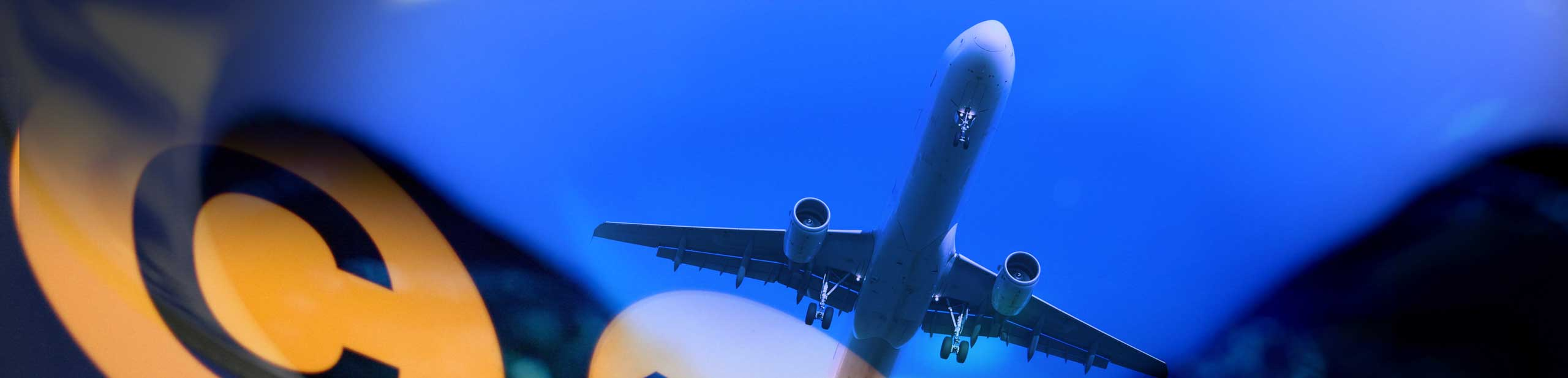Problems on statistics and probability are presented. The answers to these problems are at the bottom of the page.

1. Given the data set
4 , 10 , 7 , 7 , 6 , 9 , 3 , 8 , 9
Find
a) the mode,
b) the median,
c) the mean,
d) the sample standard deviation.
e) If we replace the data value 6 in the data set above by 24, will the standard deviation increase, decrease or stay the same?

2. Find x and y so that the ordered data set has a mean of 42 and a median of 35.
17 , 22 , 26 , 29 , 34 , x , 42 , 67 , 70 , y

3. Given the data set
62 , 65 , 68 , 70 , 72 , 74 , 76 , 78 , 80 , 82 , 96 , 101,
find
a) the median,
b) the first quartile,
c) the third quartile,
c) the interquartile range (IQR).

4. The exam grades of 7 students are given below.
70 , 66 , 72 , 96 , 46 , 90 , 50
Find
a) the mean
b) the sample standard deviation

5. Twenty four people had a blood test and the results are shown below.
A , B , B , AB , AB , B , O , O , AB , O , B , A

AB , A , O , O , AB , B , O , A , AB , O , B , A

a) Construct a frequency distribution for the data.
b) If a person is selected randomly from the group of twenty four people, what is the probability that his/her blood type is not O?

### Answers to the Above Problems

1. The given data set has 2 modes: 7 and 9
2. order data : 3 , 4 , 6 , 7 , 7 , 8 , 9 , 9 , 10 : median = 7
3. (mean) : m = (3+4+6+7+7+8+9+9+10) / 9 = 7

4. x x - m (x - m)2
4 -3 9
10 3 9
7 0 0
7 0 0
6 -1 1
9 2 4
3 -4 16
8 1 1
9 2 4
sum = 44

sample standard deviation = 2.35 (rounded to 2 decimal places)
5. The standard deviation will increase since 24 is further from away from the other data values than 6.

1. x = 36 , y = 77
1. median = 75
2. first quartile = 69
3. third quartile = 81
4. interquartile range = 81 - 69 = 12

1. mean = 70
2. sample standard deviation = 18.6 (rounded to 1 decimal place)

1.  class frequency A 5 B 6 AB 6 O 7

2. 1 - (7/24) = 17/24 = 0.71 (rounded to 2 decimal places)